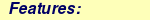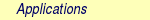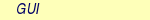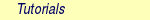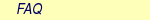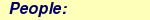MOLCAS manual:Next: 8.26 mbpt2 Up: 8. Programs Previous: 8.24 localisation

Subsections

# 8.25 LoProp

The program LoProp is a tool to compute molecular properties based on the one-electron density or transition-density and one-electron integrals like charges, dipole moments and polarizabilities. LoProp allows to partition such properties into atomic and interatomic contributions. The method requires a subdivision of the atomic orbitals into occupied and virtual basis functions for each atom in the molecular system. It is a requirement for the approach to have any physical significance that the basis functions which are classified as "occupied" essentially are the atomic orbitals of each species. It is therefore advisable to use an ANO type basis set, or at least a basis set with general contraction.

The localization procedure is organized into a series of orthogonalizations of the original basis set, which will have as a final result a localized orthonormal basis set. Note that this module does not operate with symmetry.

A static property, which can be evaluated as an expectation value, like a charge, a component of the dipole moment or an exchange-hole dipole moment, is localized by transforming the integrals of the property and the one-electron density matrix to the new basis and restricting the trace to the subspace of functions of a single center or the combination of two centers.

The molecular polarizability is the first order derivative of the dipole moment with respect to an electric field and the localized molecular polarizability can be expressed in terms of local responses. In practical terms a calculation of localized polarizabilities will require to run seven energy calculations. The first one is in the absence of the field and the other six calculations are in the presence of the field in thex,y,z axis respectively.

For a detailed description of the method and its implementation see .

## 8.25.1 Dependencies

The dependencies of the LoProp module is the union of the dependencies of the SEWARD, and the program used to perform the energy calculation, namely the SCF, MBPT2, RASSCF, or CASPT2 module. The user can also provide LoProp with a density matrix as input; then LoProp only depends on SEWARD. The one-electron transition density matrix can also be localized to compute, for example, Förster transition probabilities; then LoProp depends on RASSI to compute the transition density.

## 8.25.2 Files

The files of the LoProp module is the union of the files of the SEWARD module, and the SCF or MBPT2, or RASSCF, or CASPT2 module. An exception is if a density matrix is given as input or when a transition density matrix is localized, see below.

### 8.25.2.1 Input files

 File Contents USERDEN The density matrix given as input when the keyword USERdensity is included in the input. The density matrix should be of the following form: triangularly stored ((1,1),(2,1),(2,2),(3,1) etc.) with all off-diagonal elements multiplied by two. USERDEN1 The density matrix for a field-perturbed calculation (X = +delta) USERDEN2 The density matrix for a field-perturbed calculation (X = -delta) USERDEN3 The density matrix for a field-perturbed calculation (Y = +delta) USERDEN4 The density matrix for a field-perturbed calculation (Y = -delta) USERDEN5 The density matrix for a field-perturbed calculation (Z = +delta) USERDEN6 The density matrix for a field-perturbed calculation (Z = -delta) TOFILE The one-electron transition density matrix, which optionally can be put to disk by RASSI, see its manual pages.

### 8.25.2.2 Output files

In addition to the standard output unit LoProp will generate the following file.

 File Contents MpProp File with the input for NEMO.

## 8.25.3 Input

This section describes the input to the LoProp program. The program name is:

&LOPROP

### 8.25.3.1 Keywords

There are no compulsory keywords.

 Keyword Meaning NOFIeld The calculation is run in the absence of a field and only static properties like charges and dipole moments are computed. The default is to go beyond the static properties. DELTa The magnitude of the electric field in the finite field perturbation calculations to determine the polarizabilities. Default value is 0.001 au. ALPHa A parameter in the penalty function used for determining the charge fluctuation contribution to the polarizabilities. See eq. 17 in . The default value of 7.14 is good for small molecules (less than 50 atoms). For larger molecules, a smaller alpha (e.g. 2.0) may be needed for numerical stability. BOND Defines the maximum allowed bond length based on the ratio compared to Bragg-Slater radii. All contributions in bonds longer than this radius will be redistributed to the two atoms involved in the bond, so the the total molecular properties are left unaltered. The default value is 1.5. MPPRop Defines the maximum l value for the multipole moments written to the MpProp file. If the value specified is larger than the highest multipole moment calculated it will be reset to this value, which is also the default value. The 'MULTipoles' keyword in Seward can change the default value. EXPAnsion center Defines which points will be used as the expansion centers for the bonds. The next line must contain either 'MIDPoint' in order just to use the midpoint of the bond or 'OPTImized' in order to let LoProp move the expansion center along the bond. The latter is still highly experimental! USERdensity No density matrix is computed instead it is read as an input from the file USERDEN. This enables LoProp to obtain localized properties for densities that currently can not be computed with MOLCAS. If the keyword NOFIeld is not given, six additional files are required (USERDEN1-USERDEN6), each containing the density matrix of a perturbed calculation, see above. Observe the form of USERDEN, see above. TDENsity This keyword signals that the one-electron density matrix which is to be read comes from the TOFILE file generated by RASSI. The keyword is followed by two integers that gives number of initial and final state of the transition. For example, if it is the transition density between the first and second state which should be localized, the integers should be 1 and 2. The keyword implies NOFIeld XHOLe The exchange hole dipole moment is computed, localized and given as additional output. This quantity can be used to compute local dispersion coefficients according to Becke and Johnson. The numerical integration routine in MOLCASis used.

### 8.25.3.2 Input example

Below follows an example input to determine the localized charges, and dipole moments of acetone at the CASSCF level of theory.

&GATEWAY
Title  =  acetone
Coord  =  $MOLCAS/Coord/Acetone.xyz Basis = ANO-L-VDZP Group = C1 &SEWARD &SCF Occupation = 15 &RASSCF SPIN = 1 SYMMETRY = 1 NACTEL = 4 0 0 INACTIVE = 13 RAS2 = 4 &LOPROP NoField Expansion Center Optimized Bond = 1.5 MpProp = 2 In case the density matrix is given as input the input is of the form below (where$CurrDir is a variable defined by the user pointing to the directory where the input density is).

&Gateway
Coord  =  Water.xyz
Basis  =  6-31G*
Group  =  C1
&Seward

>>COPY  $CurrDir/Density$WorkDir/\$Project.UserDen
&LoProp
UserDensity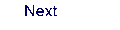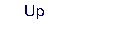Next: 8.26 mbpt2 Up: 8. Programs Previous: 8.24 localisation